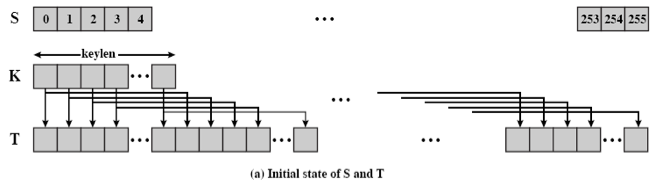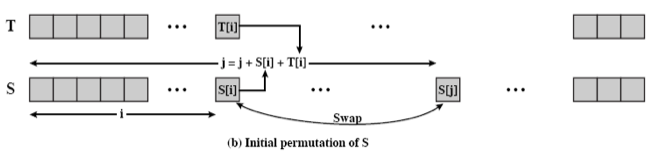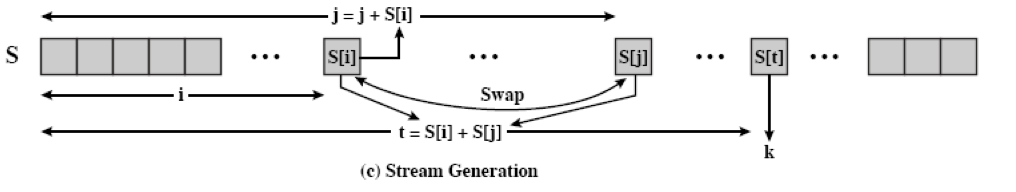# RC4¶

## basic introduction¶

Designed by Ron Rivest, RSA was originally part of RSA Security and is a patented cryptographic product. It is a byte-oriented stream cipher with a variable key length that is very simple, but it works. The RC4 algorithm is widely used in the SSL/TLS protocol and the WEP/WPA protocol.

## Basic Process¶

RC4 mainly consists of three processes

• Initialize the S and T arrays.
• Initialize the replacement S.
• Generate a key stream.

### Initializing S and T arrays¶

The code to initialize S and T is as follows

for i = 0 to 255 do

S[i] = i

T[i] = K[i mod keylen])### Initialization replacement S¶

j = 0

for i = 0 to 255 do

j = (j + S[i] + T[i]) (mod 256)

swap (S[i], S[j])### Generating a stream key¶

i = j = 0

for each message byte b

i = (i + 1) (toward 256)
j = (j + S[i]) (mod 256)

swap(S[i], S[j])

t = (S[i] + S[j]) (mod 256)

print S[t]We generally refer to the first two parts as KSA and the last part to PRGA.